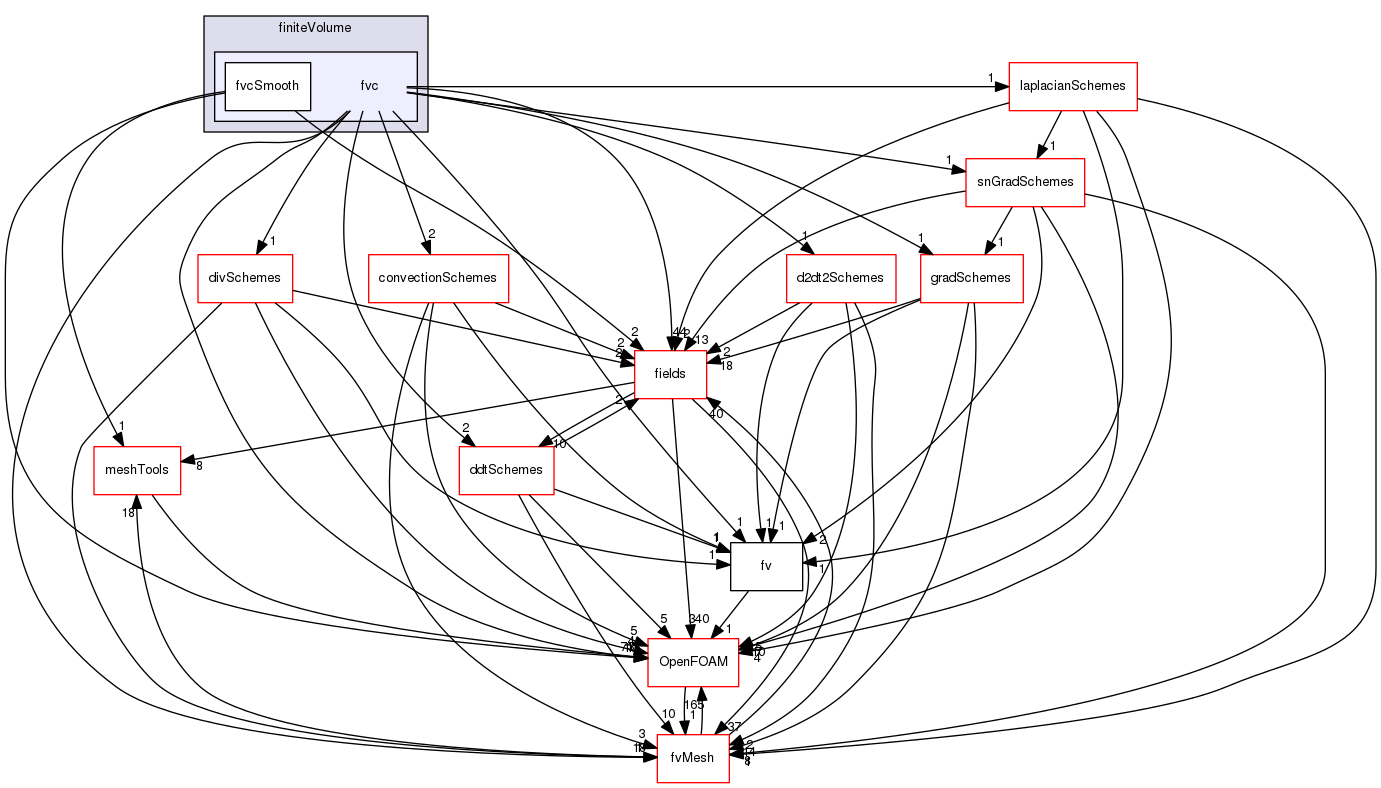The OpenFOAM Foundation
fvc Directory Reference
Directory dependency graph for fvc:## Directories

directory  fvcSmooth

## Files

file  fvc.H [code]

file  fvcAverage.C [code]

file  fvcAverage.H [code]
Area-weighted average a surfaceField creating a volField.

file  fvcCellReduce.C [code]

file  fvcCellReduce.H [code]
Construct a volume field from a surface field using a combine operator.

file  fvcCurl.C [code]

file  fvcCurl.H [code]
Calculate the curl of the given volField by constructing the Hodge-dual of the symmetric part of the gradient.

file  fvcD2dt2.C [code]

file  fvcD2dt2.H [code]
Calculate the second temporal derivative.

file  fvcDdt.C [code]

file  fvcDDt.C [code]

file  fvcDDt.H [code]
Calculate the substantive (total) derivative.

file  fvcDdt.H [code]
Calculate the first temporal derivative.

file  fvcDiv.C [code]

file  fvcDiv.H [code]
Calculate the divergence of the given field.

file  fvcFlux.C [code]

file  fvcFlux.H [code]
Calculate the face-flux of the given field.

file  fvcFluxTemplates.C [code]

Calculate the gradient of the given field.

file  fvcLaplacian.C [code]

file  fvcLaplacian.H [code]
Calculate the laplacian of the given field.

Calculate the magnitiude of the square of the gradient of the gradient of the given volField.

file  fvcMeshPhi.C [code]

file  fvcMeshPhi.H [code]
Calculate the mesh motion flux and convert fluxes from absolute to relative and back.

file  fvcReconstruct.C [code]

file  fvcReconstruct.H [code]
Reconstruct volField from a face flux field.

file  fvcReconstructMag.C [code]

file  fvcSimpleReconstruct.C [code]

Calculate the snGrad of the given volField.

file  fvcSup.C [code]

file  fvcSup.H [code]
Calculate the field for explicit evaluation of implicit and explicit sources.

file  fvcSurfaceIntegrate.C [code]

file  fvcSurfaceIntegrate.H [code]
Surface integrate surfaceField creating a volField. Surface sum a surfaceField creating a volField.

file  fvcVolumeIntegrate.C [code]

file  fvcVolumeIntegrate.H [code]
Volume integrate volField creating a volField.